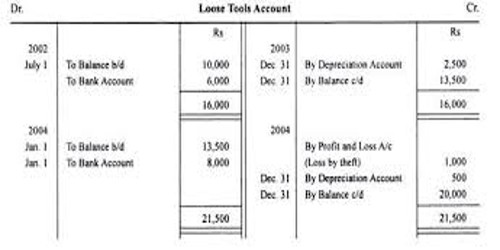# Revaluation Method of Calculating Depreciation

Revaluation Method of Calculating Depreciation

Under this method, the assets like loose tools are revalued at the end of the accounting  period and the same is compared with the value of the asset at the beginning of the year. The difference is considered as depreciation.

Revaluation = Opening balance + Purchases – Closing balance

The easiest depreciation method, but most students failed to recall the formula or are unknown of this method

Example:

If the opening balance of a loose tools account amounted to \$2,000 and during the year, the business purchased \$500 worth of loose tools and at the end of the year, the loose tools accounted to \$1,500.

Depreciation = OB + P – CB = \$2,000 + \$ 500 – \$1,500 = \$ 1,000.

This is the simplest method. With the help of experts, compare the value of the assets at the end of the year with the value at the beginning of the year. The loss on revaluation is debited to Profit and Loss Account or Depreciation Account and credited to Asset Account. This method is used for small items like livestock, loose tools, packages etc.

Merits of Revaluation Method: This method is very simple and the amount of loss can be ascertained very easily.

Demerits:

• As every year revaluation is desired it presents various problems.
• Amount of depreciation is always unequal.
• The valuation of asset is very time-consuming.